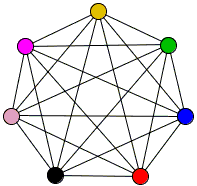# Császár Polyhedron 1

We are concerned here with a curious phenomenon of a polyhedron with no diagonals. Tetrahedron provides the simplest example. Its skeleton is the complete graph K4.Recollect the Euler polyhedral formula:

V - E + F = 2

which holds for simple polyhedrons.

Any polyhedron with no diagonals satisfies two conditions:

1. E = V(V - 1)/2,
2. 3F = 2E, because each of the faces is a triangle (lest there be a diagonal inside the face).

Substituting these into Euler's formula gives V - V(V - 1)/2 + V(V - 1)/3 = 2. The resulting quadratic equation has two roots V = 3 (the triangle with two faces - inside and outside) and V = 4 (the tetrahedron).

However there are other polyhedrons with no diagonals. These ones could not be simple. For the figures with h holes/tunnels the Euler formula has to be modified:

V - E + F = 2 - 2h.

However, regardless of the number of holes any figure with no diagonals is bound to satisfy the two properties above, thus again substituting the two but now into the extended Euler formula we obtain V - V(V - 1)/2 + V(V - 1)/3 = 2 - 2h. This gives a formula for h in terms of V, the number of vertices:

h = (V - 3)(V - 4)/12.

Now, for V = 3 and V = 4, h = 0 as it should. The next integer value for h is obtained with V = 7. For V = 7, E = 12(12 - 1)/2 = 21, F = 2·21/3, h = 1. This means a polyhedron with 7 vertices, 21 edges, 14 faces, and 1 hole. The latter means that, provided such a polyhedron exists, it is a toroid. This also means that the skeleton of such a polyhedron must be the complete graph K7 on 7 verticesThe polyhedron that answers all these requirement indeed exists and was discovered in the late 1940s by Ákos Császár [Gardner, p. 139-152].

### If you are reading this, your browser is not set to run Java applets. Try IE11 or Safari and declare the site https://www.cut-the-knot.org as trusted in the Java setup.What if applet does not run?

Drag the mouse to rotate the prism. Use the right button to remove and put back individual faces.

(Acknowledgement: I have learned most of Java details from the implementation by Meiko Rachimow. I found the geometry of the solid at the software3d online forum.)

### References

1. M. Gardner, Time Travel and Other Mathematical Bewilderments, W.H.Freeman and Co., NY, 1988.• Right Pentagonal Prism
• Square Pyramid
• Right Triangular Prism
• Twisted Triangular Prism
• Tetrahedron: an Interactive Model
• Octahedron: an Interactive Model
• Cube: an Interactive Model
• Icosahedron: an Interactive Model
• Dodecahedron: an Interactive Model
• Three Pyramids are Better Than Two
• Cube In Octahedron
• Octahedron In Cube
• Octahedron In Tetrahedron
• Tetrahedron In Cube
• Icosahedron In Cube
• Great Stellated Dodecahedron
• Lennes' Polyhedron
• Triangulated Dinosaur
• Volumes of Two Pyramids
• Császár Polyhedron 1
• Császár Polyhedron 4
• Szilassi Polyhedron
• Dissection of a Square Pyramid
•